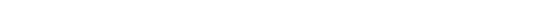NEWS NLREG has been selected as the "Editor"s Pick" by SoftSeek.NLREG is in use at hundreds of universities, laboratories, and government agencies around the world (over 20 countries). For a list of a few organizations using NLREG click here. If you have categorical variables, you may want to use a Decision Tree to model your data. Check out the DTREG Decision Tree Builder. You also should check out the News Rover program that automatically scans Usenet newsgroups, downloads messages of interest to you, decodes binary file attachments, reconstructs files split across multiple messages, and eliminates spam and duplicate files.## Circular Regression -- Fit a Circle to Data Points

There is a class of nonlinear regression problems that can be best expressed by omitting the dependent variable (i.e., the variable on the left of the equal sign). To understand what this means, first consider the normal regression case with a dependent variable. For each observation, the function is evaluated, and the computed function value is subtracted from the corresponding value of the dependent variable for that observation. This residual value is then squared and added to the other squared residual values. The goal is to minimize the total sum of squared residuals.

In the case where the dependent variable is omitted, the function is computed for each observation and the value of the function is squared (i.e., it is treated as the residual) and added to the other squared values. The goal is to minimize the sum of the squared values of the function. Thus, for a perfect fit the computed value of the function for every observation would be zero.

To perform this type of analysis omit the dependent variable and equal sign from the left side of the function specification in your NLREG program.

As an example of this type of analysis, consider the problem of fitting a circle to a set of points that form a roughly circular pattern (i.e., a "circular regression"). Our goal is to determine the center point of the circle (Xc,Yc) and the radius (R) which will make the circle best fit the points so that the sum of the squared distances between the points and the perimeter of the circle is minimized (the points are as close to the perimeter of the circle as possible).

For this problem, we have three parameters whose values are to be determined: Xc, Yc, and R. There will be one data observation for each point to which the circle is being fitted. For each point there are two variables, Xp and Yp, the X and Y coordinates of the point's position.

Since our goal is to minimize the sum of the squared distances from the points to the perimeter of the circle, we need a function that will compute this distance for each point. If the center of the circle is at (Xc,Yc) and the position of a point is (Xp,Yp) then, from the theorem of Pythagoras, we know the distance from the center to the point is

```  sqrt((Xp-Xc)^2 + (Yp-Yc)^2)
```
But we are interested in the distance from the perimeter to the point. Since the radius of the circle is R, the distance from the perimeter to the point (along a straight line from the center to the point) is
```  sqrt((Xp-Xc)^2 + (Yp-Yc)^2) - R
```
That is, the distance from the perimeter to the point is equal to the distance from the center to the point less the distance from the center to the perimeter (the radius). The distance will be positive or negative depending on whether the point is outside or inside the circle, but this does not matter since the value is squared as part of the minimization process.

The NLREG statements for this analysis are as follows:

```Title "Fit circle to group of points";
Variable Xp;                    // X coordinate of point
Variable Yp;                    // Y coordinate of point
Parameter Xc;                   // X position of circle center
Parameter Yc;                   // Y position of circle center
Parameter R;                    // Radius of circle
Function sqrt((Xp-Xc)^2 + (Yp-Yc)^2) - R;
Data;
[ data goes here ]
```
Note that there is no dependent variable or equal sign to the left of the function. NLREG will determine the values of the parameters Yp, Yc, and R such that the sum of the squared values of the function (i.e., the sum of the squared distances) is minimized.